# Convert Text String to Date – Excel & Google Sheets

This tutorial will demonstrate how to convert a text string to a date in Excel and Google Sheets.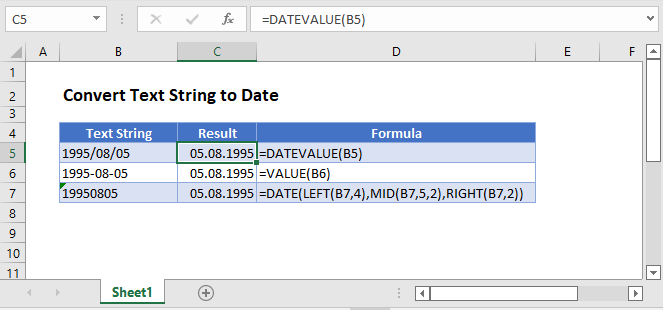## Convert Text String to Date

If you have a date in Excel stored as text, you will not be able to use the date in any calculations. However, you can convert a date stored as text to an actual date in Excel by using the DATEVALUE Function.

``=DATEVALUE(B3)``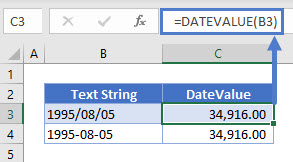Dates in Excel are stored as a value representing the number of days since the first day of Excel.

The first day of Excel is the imaginary date of January 0, 1900. So the date 05/08/1995 is stored as the number 34,916.

To display the serial number as a date, simply change the number formatting to date.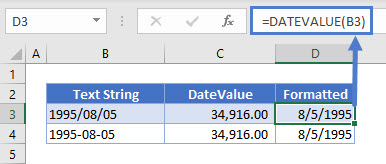The VALUE Function will work in the same way

``=VALUE(B3)``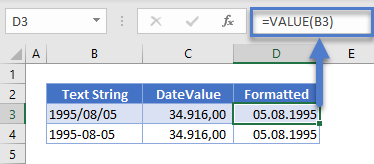## Convert Text ‘yyyymmdd’ to Date

There might be a time that you need to convert a number or a text string in the format ‘yyyymmdd’ to a date. To do this, you will need to use the DATE function, in conjunction with the LEFT, MID and RIGHT Functions.

``=DATE(LEFT(B3,4), MID(B3,5,2), RIGHT(B3,2)``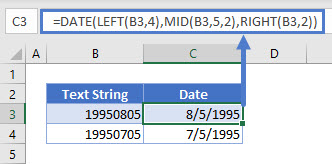### The LEFT Function

The LEFT function will return characters on the left of your text string, in this case we have selected to return 4 characters.

### The MID Function

The MID Function will return middle characters in your text string.  The starting middle character is indicated by the second argument (eg: 5), and the length of the string is indicated by the third arguments (eg: 2).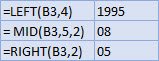### The RIGHT Function

The RIGHT function will return the characters to the right of your text string, in this case we have selected to return 2 characters.

### The DATE Function

The DATE function will then take the 3 extracted values and combine them to become a valid date, eg: DATE(1995,08,05) where the values are replaced by the LEFT, MID and RIGHT functions.

### Easy Excel Automation## Convert Text String in Google Sheets

The DATEVALUE and VALUE Function work the same way in Google Sheets as they do in Excel.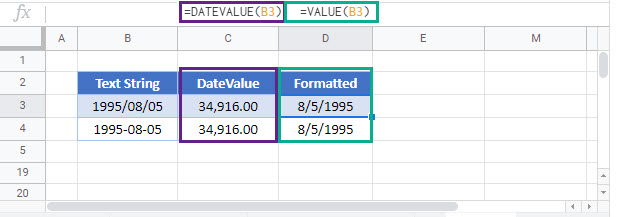The DATE, LEFT, MID and RIGHT Functions also work the same in Google Sheets as they do in Excel.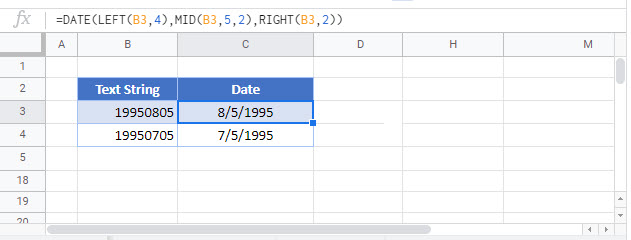### Excel Practice Worksheet

Practice Excel functions and formulas with our 100% free practice worksheets!